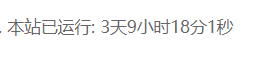1.在模板想插入的位置（比如页脚footer.php）中插入以下代码：

``````<span id="runtime_span"></span>
<script type="text/javascript">function show_runtime(){window.setTimeout("show_runtime()",1000);X=new
Date("05/23/2020 8:00:00");
Y=new Date();T=(Y.getTime()-X.getTime());M=24*60*60*1000;
a=T/M;A=Math.floor(a);b=(a-A)*24;B=Math.floor(b);c=(b-B)*60;C=Math.floor((b-B)*60);D=Math.floor((c-C)*60);
runtime_span.innerHTML="本站勉强运行: "+A+"天"+B+"小时"+C+"分"+D+"秒"}show_runtime();</script>
``````

2.修改初始网站建设时间：

``````Date("05/23/2020 8:00:00")
``````